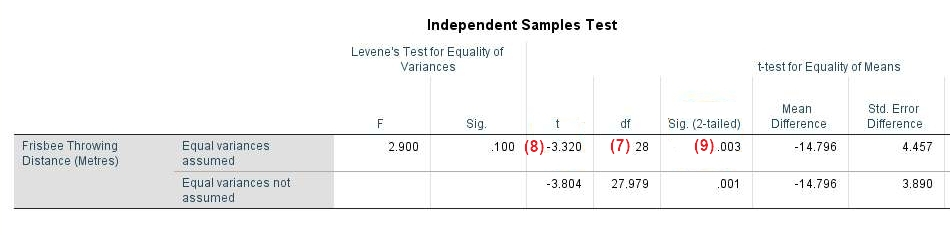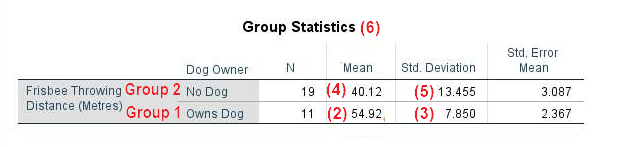# Reporting an Independent Samples t-Test from SPSS in APA Style

In this tutorial, we show you how to report an independent samples t-test from SPSS in APA style.

First, we provide a template that you can use to report your independent samples t-test in APA style. Then we show you how to populate this template using the SPSS output from your own t-test. Finally, we show you an example of an independent samples t-test report written using our template.

## Template for Reporting an Independent Samples t-Test in APA Style

A template for reporting your independent samples t-test is below.  Replace the [blue bold text in square brackets] with information from your own t-test.  The (red numbers in parentheses) correspond to numbers on the screenshots of SPSS output below and/or to our notes and tips for writing your report (also below).

Note: To keep this tutorial simple, we make the assumption that you conducted a “two-tailed” test.  This means that you conducted an independent samples t-test to evaluate whether your dependent variable differed in any direction between the two groups that you compared.

### The Template

An independent samples t-test was performed to evaluate whether there was a difference between the [dependent variable] of [group 1 of independent variable (IV)] and [group 2 of IV].

The results indicated that (1) there was no significant difference between the [dependent variable] of [group 1 of IV] (M = [mean for group 1 of IV (2)], SD = [standard deviation for group 1 of IV (3) ]) and [group 2 of IV] (M = [mean for group 2 of IV (4)], SD = [standard deviation for group 2 of IV (5)]) OR [group 1 of IV] (M = [mean for group 1 of IV (2)], SD = [standard deviation for group 1 of IV (3)]) had significantly higher/lower (6) [dependent variable] than [group 2 of IV] (M = [mean for group 2 of IV (4)], SD = [standard deviation for group 2 of IV (5)]) t([df (7)]) = [value of t (8)]p = [value of p (9)].

## Populating the Independent Samples t-Test APA Template with Your SPSS Output

The screenshots below are from an independent samples t-test that we performed on a fictitious data set to evaluate whether there was a difference between the frisbee throwing distances of dog owners and people who did not own dogs.  The numbers on the screenshots correspond to numbers in the APA template above.  Take the corresponding values from your own SPSS output and use them to populate our template.

Note that the APA Style Guide states that: (a) the first line of each paragraph should be indented 0.5 inches from the left margin; and (b) the text should be double-spaced.

(1) Use the text highlighted yellow if there was no significant difference between the two groups in your study (dog owners and non-dog owners in our example)  Use the text highlighted gold if there was a significant difference between the groups.  The difference is significant if the p value in the “Sig. (2-tailed)” column of the “Equal variances assumed” row of the “Independent Samples Test” table ((9) in the screenshot below) is less than or equal to the alpha level you selected for your test.  Selecting an alpha level of .05 is typical.(2) and (4) Report the means for each group to two decimal places.

(3) and (5) Report the standard deviations for each group to two decimal places.

(6) Check the “Group Statistics” table to determine which group has the highest (or lowest) mean score on the dependent variable.  In our example, the mean frisbee throwing distance was higher for dog-owners (54.92 metres) than for non dog owners (40.12 metres).  Select an appropriate adjective to compare the two groups in your study, e.g., highest, lowest, fastest, etc.

(7) Report the degrees of freedom (df) from the “Equal variances assumed” row of the “Independent Samples Test” table in your SPSS output.

(8) Report the value of t from the “Equal variances assumed row of the “Independent Samples Test” table to two decimal places.  If your t value is smaller than +1.00/-1.00, add a leading zero (e.g., write 0.87 rather than .87).  If your t value is negative, you can report it as a positive value.  So, in our example, we report t as 3.32 rather than -3.32.

(9) Report the exact p value to two or three decimal places as per the “Sig. (2-tailed)” column of the “Equal variances assumed” row of the “Independent Samples Test” table.  If the value is .000, however, report it as < .001.  You should not add a leading zero to your p value.

We encourage you to modify the wording of the template if doing so allows you to communicate your results more clearly.

## Example of an Independent Samples t-Test Report in APA Style

An independent samples t-test was performed to evaluate whether there was a difference between the [frisbee throwing distances] of [people who owned dogs] and [people who did not own dogs].

The results indicated that [dog owners] (M = [54.92], SD = [7.85]) had significantly greater [frisbee throwing distances] than [non dog owners] (M = [40.12], SD = [13.46]) t() = [3.32]p = [.003].

**************

That’s it for this tutorial.  You should now be able to report the results of an independent samples t-test from SPSS in APA style.

***************